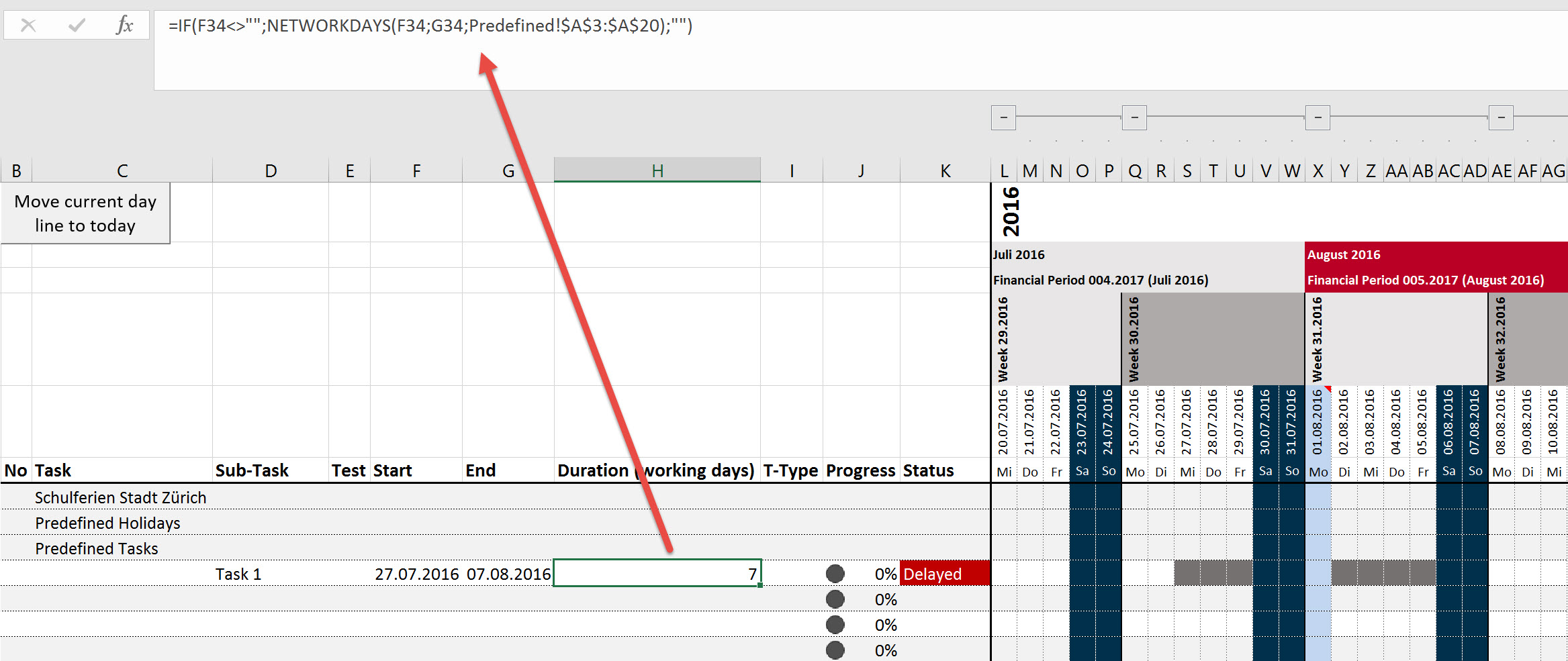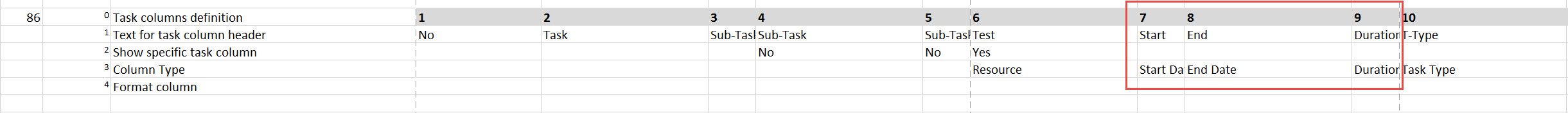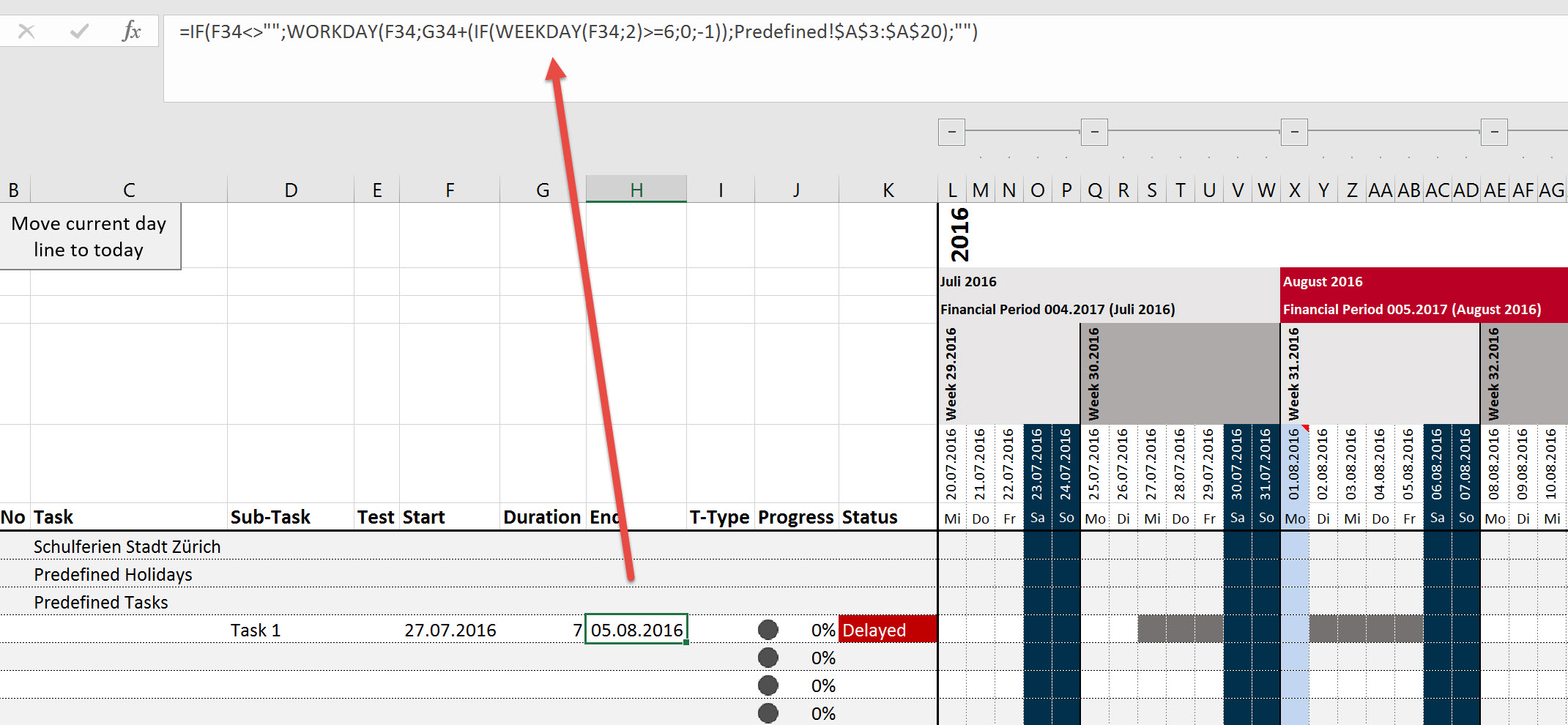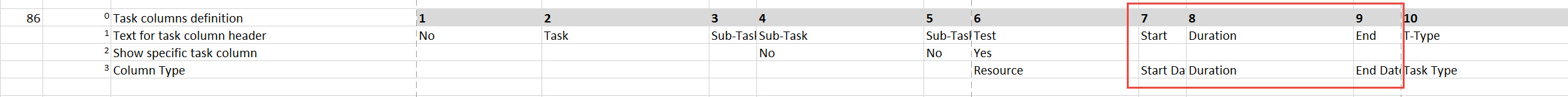# Setting description

ID 00087
Name Start / End / Duration - Calculation Definition
Available values
 Duration is calculated by start- and end date End date is calculated by start date and duration
Default value End date is calculated by start date and duration
Description You can define if the duration should be calculated or the end date.
Prerequisite The calculation depends also on the setting 00070 - Workdays (Duration) Definition.

In this example the setting 00070 - Workdays (Duration) Definition has been set to "Only Weekdays (Excl. Public Holidays) to illustrate the different impact. With this setting public holidays and weekends are not taken in account for the workdays calculation.

In this example we defined the August 1st as a public holiday and therefore this day is not calculated as a working day.

# Duration is calculated by start- and end date

If you work with this setting, then you have to enter for each task the start and the end date. In the duration column you see the formula (can be different - depends on setting 00070 - Workdays (Duration) Definition) which calculates the workdays based on the start and end date.

In the below task the end date is a Sunday - August 07th. Therefore the overall duration will be 7 days.## Recommendation

If you use this setting, we recommend to adjust the 00086 - Task columns definition setting as follow - so you can enter the end date direct after the start date.# End date is calculated by start date and duration

If you work with this setting, then you have to enter for each task the start and the duration. In the end column you see the formula (can be different - depends on setting 00070 - Workdays (Duration) Definition) which calculates the end date based on the start and duration.

In the below task the duration is entered as 7 workdays - therefore the end date is the August 5th. This is the difference for the above example where we entered as end date August 7th (Sunday) and also 7 workdays were calculated.## Recommendation

If you use this setting, we recommend to adjust the 00086 - Task columns definition setting as follow - so you can enter the duration direct after the start date.